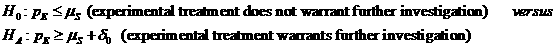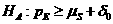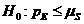# Bayesian Phase II Design

Hypothesis:Data Input: (Help) (Example)

 Input Results W90 μS d0 cE Nmax Numstage (1-3) Prior Distribution Enthusiastic Skeptical Flat

*Since the calculation takes some times, if the Web Browser shows the pop-up window to ask you to stop the script, please click "No" until the results have shown.

Note:

 Variables Descriptions W90 The width of the 90% probability interval from the 95th to 5th percentiles μS Mean response rate of standard therapy d0 Fixed targeted improvement cE Concentration parameter of experimental treatment 2 ≦ cE ≦ 10 Nmax Maximum number of patients to be recruited Numstage Maximum number of stages for patient recruitment ni Number of patients to be accrued in stage i Li Lower decision boundary in stage i Ui Upper decision boundary in stage i Enthusiastic Enthusiastic prior distribution mE = mS + d0 Skeptical Skeptical prior distribution mE = mS Flat Flat prior distribution mE = 0.50

Help Aids

Application:   The main goal of Phase II clinical trials is to identify the therapeutic efficacy of new treatments. The formulation and implementation of a Bayesian design is proposed for planning and monitoring a single-arm Phase II clinical trial.

Procedure:

1. Enter

a)   value of W90, the width of the 90% probability interval from the 95th to 5th percentiles

b)   value of μS, the mean response rate of standard therapy

c)   value of d0, the fixed targeted improvement

d)   value of cE, the concentration parameter of experimental treatment

e)   value of Nmax, the maximum number of patients to be recruited

f)   value of Numstage, the maximum number of stages for patient recruitment.

1. Click the button “Calculate” to obtain

a)    value of ni, the number of patients to be recruited in stage i

b)    value of Li, the lower decision boundary in stage i

c)   value of Ui, the upper decision boundary in stage i

Assumption:

1.      Assume that a Phase I trial of the experimental treatment regimen has been completed, from which the proper dosages yielding acceptable toxicity have been established.

2.      A maximum of K=1,2 or 3 tests is adopted in the calculator, since more frequent testing would not seem to be necessary in most Phase II trials.

3.      Due to the very limited number of patients who are eligible for a given Phase II study, and also to the large number of treatment regimens generally awaiting Phase II trials, the sample size N for a given trial is frequently fixed in advance.

Theory:

Hypotheses

The null and alternative hypotheses for the Bayesian design are as follows:

H0: pE £ mS (experimental treatment does not warrant further investigation)  versus

H1: pE ³ mS + d0 (experimental treatment warrants further investigation),

where pE is the true response probabilities of the experimental treatment, mS is the mean response rate of the standard treatment, and d0 is the fixed targeted improvement.

The Bayesian procedure for designing Phase II clinical trials uses the concept the prior evidence can be summarized as prior probabilities which are modified by the experimental data to yield revised beliefs or posterior probabilities. A clinician can summarize the previous information and knowledge about a treatment response probability q in order to construct a probability distribution for q. This distribution is called a prior distribution because it represents the relative likelihood that the true value of q lies in various regions of some specified parameter space prior to the observations of any values of the data.

Let qS and qE represent the patient response rates for the standard and experimental treatments, respectively. Let Xn be the number of treatment responses after n patients have been recruited. The Bayesian design recruits nmin = 10 subjects and applies the decision rules discussed below to determine whether the trial should be terminated at the first stage or if recruitment should continue to stage n + 1 and another patient added to the study. This procedure is repeated until nmax = 65 subjects have been recruited. The value nmin = 10 is chosen because it is the conventional minimum sample size and the value of nmax = 65 is chosen because it is often impractical to have a larger sample size for Phase II studies.

Prior Distributions

There are several choices of prior distributions to be used for the response probability qE of the experiment treatment. The prior distributions used are beta(a, b) distributions with p.d.f.  P{q} µ qa-1 (1-q)b-1, where 0 < q < 1 and a, b > 0.

All prior distributions for the standard treatment can be parameterized in terms of the mean response rate mS = aS / (aS+bS) and W90 = the width of the 90% probability interval from the 95th to 5th percentiles, where S represents the standard therapy.  The clinician is asked to specify mS and W90 so that aS and bS may be found.

All prior distributions for the experimental treatment can be parameterized in terms of the mean response rate mE = aE / (aE+bE) and the concentration parameter cE (= aE+bE), where E represents the experimental treatment.  The clinician must specify mE and cE so that aE and bE can be found.  Note that 2 £ cE £ 10 so that the dispersion of the prior is no larger than that of the uniform (0, 1) distribution and no smaller than that of the posterior corresponding to a small pilot study of the experimental treatment.

The enthusiastic prior distribution assumes that the mean response rate of the experimental treatment is equal to a improvement over that of the standard treatment, i.e. mE = mS + d0.  Enthusiastic priors are used in situations where the experimental treatment is thought not to be an improvement over the standard treatment, or when the treatment is showing negative results.  If the treatment fails to prove promising using an enthusiastic prior, then there is overwhelming evidence to support the decision that the treatment is not promising.

The skeptical prior distribution assumes that the mean response rate of the experimental treatment is equal to that of the standard treatment, i.e. mE = mS.  Skeptical priors are used in situations where the experimental treatment is believed to be promising, or when the treatment is showing positive results.  If the results prove the experimental treatment is promising, then this would be extremely convincing evidence to support the decision that the treatment is promising.  Hence, the Bayesian design can incorporate the skeptical opinions about a treatment to yield overwhelming evidence about the treatment.

The flat prior distribution assumes mE = 0.50 and cE = 2.  The flat prior is used in situations where little is known about the efficacy of the experimental treatment.  The rationale for using uninformative priors is that inferences are dominated by the observed data rather than depending on a clinicians knowledge about the experimental treatment.  The flat prior has a uniform (0, 1) distribution, so it treats all true treatment response probabilities equally.  The weakly uninformative prior distribution assumes mE = 0.50 and cE = 10.  This may also be used in situations where little is known about the experimental treatment.  The weakly uninformative prior has dispersion like that of the posterior corresponding to a small pilot study of the experimental treatment.

The likelihood is from a binomial (n, qE) distribution with p.d.f.

P{Xn | qE} µ qEXn (1-qE) n - Xn.

The posterior p.d.f. for qE is a beta (qE; aE + Xn, bE + n - Xn) distribution with p.d.f.

P{qE | Xn} µ P{qE} P{Xn | qE}= qEXn + aE - 1 (1 - qE)n - Xn + bE – 1.

Decision Boundaries

The stopping rules are based on probabilities from the posterior distributions of treatment differences. Let pU and pL be defined as predetermined probabilities corresponding to the upper and lower decision boundaries respectively. For this, pL = 0.05 and pU = 0.95.

Un = smallest integer x such that P{qE > qS | Xn = x} ³ pU (experiment treatment declared promising), and

Ln  = largest integer x such that P{qE > qS + d0 | Xn = x} £ pL (experiment treatment declared not promising).

The value of Un is the smallest value of x at which the probability that the response probability of the experimental treatment is greater than that of the standard treatment given the observed number of treatment successes is at least pU. Any value greater than Un would give a probability greater than pU, which implies that the treatment is promising. The value of Ln is the largest value of x at which the probability that the response probability of the experimental treatment is greater than that of the standard treatment plus some targeted improvement given the observed number of treatment successes is at most pL.  Any value less than Ln would give a probability less than pL, which implies that the treatment is not promising.  These criteria are used to generate decision boundaries and stopping criteria.

Note that P{qE > qS + d0 | Xn = x}, like the probability of any random variable with a continuous distribution, can be expressed in terms of an integral.

P{qE > qS + d0 | Xn = x out of n patients} = 1 - P{qE £ qS + d0 | Xn = x out of n patients}

= E[1 - ò0qs + do ¦(z;aE + x, bE + n - x)dz]

= E1 - F(z;aE + x, bE + n - x) |0qs + do]

= E[1 - {F(qS + d0;aE + x, bE + n - x) - 0}]

= ò01 - do [1 - F(z + d0;aE + x, bE + n - x)] ¦(z; aS, bS)dz, where

E[·] is the expected value with respect to the distribution of qS,

¦(·; aS, bS)is the p.d.f. of the prior distribution for the standard treatment, and

F(·;aE + x, bE + n - x)is the c.d.f. of the posterior distribution for the experimental treatment.

Stopping Criteria

At stage n, the decision rules are:

·         If Xn ³ Un, then terminate the trial and reject H0, i.e. declare treatment promising

·         If Xn £ Ln, then terminate the trial and reject HA, i.e. declare treatment not promising

·         If Ln < Xn < Un and n < nmax, then continue to stage n + 1

The trial is declared inconclusive if Xn has not hit a decision boundary by n = nmax.  This procedure also provides for continual sequential monitoring.

Example:

Since Phase I studies indicate that the experimental regimen is somewhat more toxic than the standard, in phase II clinical trial, it is decided to set W90=0.2, μS=0.2, cE=2, d0=0.2, the maximum 25 patients accrual in 2 stages (Nmax=25, Numstage=2). Enthusiastic prior distribution is used. The number of patients to accrue in stage 1 is 15 (n1=15) and in the last stage (stage 2), 25 patients are tests (n2=10). The lower and upper decision boundary in stage 1 is 2 and 7 respectively (L1=2, U1=7), it means that if the number of treatment response in stage 1 is less than or equal to 2, then stop the study and reject the alternative hypothesis, if the number of treatment response in stage 1 is greater than or equal to 7, then stop the study and reject the null hypothesis, if the number of treatment response in stage 1 is greater than 2 and less than 7, then continue to stage 2 to recruit 10 more patients. The lower and upper decision boundary in stage 2 is 5 and 10 respectively (L2=5, U2=10), it means that if the number of treatment response in stage 2 is less than or equal to 5, then stop the study and reject the alternative hypothesis. I f the number of treatment response in stage 2 is greater than or equal to 10, then stop the study and reject the null hypothesis. If there is no conclusion about the trial in the last stage, then the trial is declared inconclusive.

Reference: Simon, Thall (1994) Biometrics 50: 337-349